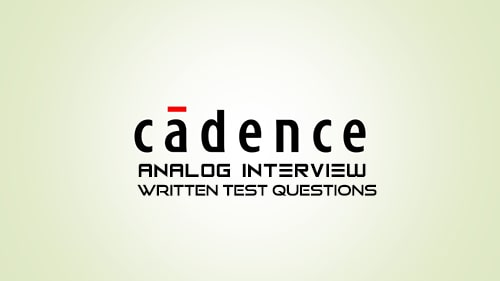You are here
Home > Analog >The written test analog questions were asked in the cadence interview process. RC circuits and Op-Amp questions are important.
The interview process started in the early morning in their company building. We were around 12 students from the same college.

The HR alone met us. The cadence HR took two rounds of written tests Digital and Analog. The digital written test round was a bit easy. Verilog and basic digital circuit design based on problem statement questions were there. And the analog written test was tough, so many in-depth questions were there. Knowledge network theory and series RLC circuits were a must need.

The shortlisted students for both sections separately. Few aspirants were selected for both analog and digital profiles. Then they conducted a personal interview for the candidates who were shortlisted after the lunch hour.

There were around three to four interview rounds both for analog and digital, it lasted till the evening at 7 pm. The next day they announced the results. They have taken two guys one for analog and one for the digital profile. A few of the analog questions which were asked are listed below.

1. In the given circuit below, What is the value of voltage Vout?2. For the given circuit below calculate Vout.3. The circuit below is powered up at t = 0; Assuming zero initial conditions, calculate what is the value of Vout at a) t = 0 and b) t = infinity.4. In the following figure, what is the voltage across the capacitor C, whose value is 1pF?5. Initially, C1 has a charge of 1C and 1V across the plates. C2 is a 1F capacitor with no charge. The switch is closed at t = 0, what is the total energy dissipated on the register R?7. Draw the circuit to generate the following sequences
1. 11_01_10_11
2. 00_10_01_00
8. Find the value of Vout at the given input conditions below
1. V = 1V
2. V = (100u +sin(wt))V | (1/wc) << 100 Ohm
3. V = Sin(wt)V | (1/wc) << 100 Ohm9. Identify the terminals of the Op-Amp such that the circuit has DC negative feedback for the following cases1. R1 = 3R and R2 = R
2. R1 = R and R2 = 2R
3. Also find out Vout/Vin as a function of R1, R2, and R. by assuming DC negative feedback.

10. For the given following circuit, draw the Vr verses Vi characteristics. Given that Vd = 0.7V, RC = 1us.11. Find the steady-state output Vout across parallel RC combinations. Vi = Vp cos (t/RC).12. Find the frequency response Vout verses, Vin.13. Assume that the switch was closed before t = 0. The switch SW1 is opened at t = 0; Find the voltage Vout at t = 0+.Be ready with the BTECH and MTECH project and Prepare well for all of the above-related topics. Mainly focus much on the RC circuits problems and problems based on Op-Amps. If you want solutions to the above questions, you can contact me.